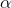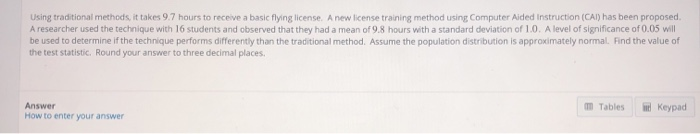Question

Using traditional methods, it takes 11.2 hours to receive a basic flying license. A new license training method using Computer Aided Instruction (CAI) has been proposed. A researcher used the technique with 16 students and observed that they had a mean of 11.4 hours with a standard deviation of 1.6. A level of significance of 0.10 will be used to determine if the technique performs differently than the traditional method. Assume the population distribution is approximately normal. Find the value of the test statistic. Round your answer to three decimal places.

Solution := 11.2= 11.4

s = 1.6

n = 16= 0.10

Test statistic = t =

= (-) / s /n

= (11.4 -11.2 ) / 1.6/16

Test statistic = t = 0.500

#### Earn Coins

Coins can be redeemed for fabulous gifts.

Similar Homework Help Questions

Using traditional methods, it takes 11.2 hours to receive a basic flying license. A new license training method using Computer Aided Instruction (CAI) has been proposed. A researcher used the technique with 16 students and observed that they had a mean of 11.41. hours with a standard deviation of 1.61. A level of significance of 0.1 will be used to determine if the technique performs differently than the traditional method. Assume the population distribution is approximately normal. Find the value...

Using traditional methods, it takes 10.0 hours to receive a basic flying license. A new license training method using Computer Aided Instruction (CAI) has been proposed. A researcher used the technique with 16 students and observed that they had a mean of 10.2 hours with a standard deviation of 1.6. A level of significance of 0.05 will be used to determine if the technique performs differently than the traditional method. Assume the population distribution is approximately normal. Find the value...

Using traditional methods, it takes 8.6 hours to receive a basic flying license. A new license training method using Computer Aided Instruction (CAI) has been proposed. A researcher used the technique with 16 students and observed that they had a mean of 8.8 hours with a standard deviation of 1.0. A level of significance of 0.1 will be used to determine if the technique performs differently than the traditional method. Assume the population distribution is approximately normal. Find the value...

Using traditional methods, it takes 9.09.0 hours to receive a basic flying license. A new license training method using Computer Aided Instruction (CAI) has been proposed. A researcher used the technique with 1717 students and observed that they had a mean of 9.29.2 hours with a standard deviation of 1.11.1. A level of significance of 0.10.1 will be used to determine if the technique performs differently than the traditional method. Assume the population distribution is approximately normal. Find the value...

Using traditional methods, it takes 11.9 hours to receive a basic flying license. A new license training method using Computer Aided Instruction (CAI) has been proposed. A researcher used the technique with 9 students and observed that they had a mean of 12.2 hours with a standard deviation of 1.5. A level of significance of 0.05 will be used to determine if the technique performs differently than the traditional method. Assume the population distribution is approximately normal. Find the value...

Using traditional methods, it takes 96 hours to receive a basic flying license. A new license training method using Computer Aided Instruction (CAI) has been proposed. A researcher used the technique with 150 students and observed that they had a mean of 95 hours. Assume the standard deviation is known to be 88. A level of significance of 0.05 will be used to determine if the technique performs differently than the traditional method. Find the value of the test statistic....

Using traditional methods, it takes 104 hours to receive a basic flying license. A new license training method using Computer Aided Instruction (CAI) has been proposed. A researcher used the technique with 80 students and observed that they had a mean of 106 hours. Assume the standard deviation is known to be 7. A level of significance of 0.02 will be used to determine if the technique performs differently than the traditional method. Find the value of the test statistic....

Using traditional methods, it takes 104 hours to receive a basic flying license. A new license training method using Computer Aided Instruction (CAI) has been proposed. A researcher used the technique with 60 students and observed that they had a mean of 102 hours. Assume the standard deviation is known to be 8. A level of significance of 0.05 will be used to determine if the technique performs differently than the traditional method. Find the value of the test statistic....

Using traditional methods, it takes 92 hours to receive a basic flying license. A new license training method using Computer Aided Instruction (CAI) has been proposed. A researcher used the technique with 70 students and observed that they had a mean of 93 hours. Assume the standard deviation is known to be 6. A level of significance of 0.05 will be used to determine if the technique performs differently than the traditional method. Find the value of the test statistic....

• ### using traditional methods, it takes 9.7 hours to receive a basic flying Using traditional methods, it...using traditional methods, it takes 9.7 hours to receive a basic flying Using traditional methods, it takes 9.7 hours to receive a basic flying license. A new license training method using Computer Aided Instruction (CAI) has been proposed. A researcher used the technique with 16 students and observed that they had a mean of 9.8 hours with a standard deviation of 1.0. A level of significance of 0.05 will be used to determine if the technique performs differently than the...